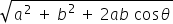science
Easy

Question

# If a vector has zero magnitude, then the vector is called as

## Unit vectorOrthogonal vectorNegative vectorNull vectorHint:

## The correct answer is: Null vector

### If the vector has zero magnitude, then the vector is called as null vector. It is directionless.

Null vector is a directionless vector whose magnitude is zero and the resultant vector of two or more equal vectors that are acting opposite to each other.

science

### Parallelogram law is used when _______

In parallelogram law of vector addition, it says that if we place two vectors so they have the same initial points(the tails are connected) and then complete the vectors into a parallelogram, then the sum of the vectors is directed diagonal that starts at the same point as the vectors.

### Parallelogram law is used when _______

In parallelogram law of vector addition, it says that if we place two vectors so they have the same initial points(the tails are connected) and then complete the vectors into a parallelogram, then the sum of the vectors is directed diagonal that starts at the same point as the vectors.

science

science

### Two vectors started from the origin and moved to the east and north direction 5 m, 7 m, respectively, and the angle between the vectors is 90 degrees, then find the resultant of the vectors.science

### Two vectors started from the origin and moved to the east and north direction 10 m, 15 m, respectively, and the angle between the vectors is 30 degrees, then find the resultant of the vectors.

If two vectors A and B with magnitude a and b respectively are given and the angle between them is θ Then the magnitude of the Resultant of A and B is R =### Two vectors started from the origin and moved to the east and north direction 10 m, 15 m, respectively, and the angle between the vectors is 30 degrees, then find the resultant of the vectors.

If two vectors A and B with magnitude a and b respectively are given and the angle between them is θ Then the magnitude of the Resultant of A and B is R =science

science

### If a vector has magnitude 1, then the vector is called asscience

science

science

### The displacement in a closed curve isscience

science

science

### The angle in the triangle law is represented byscience

science

science

### If the vectors are of the same magnitude and same direction, they are said to be

Two vectors are said to be equal if they have same magnitude and direction, regardless of the positions of their positions of their initial points.

### If the vectors are of the same magnitude and same direction, they are said to be

Two vectors are said to be equal if they have same magnitude and direction, regardless of the positions of their positions of their initial points.#### With Turito Foundation.#### Get an Expert Advice From Turito.